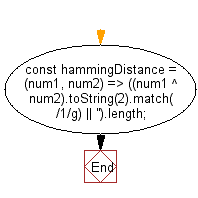# JavaScript: Calculate the Hamming distance between two values

## JavaScript fundamental (ES6 Syntax): Exercise-219 with Solution

Write a JavaScript program to calculate the Hamming distance between two values.

• Use the XOR operator (^) to find the bit difference between the two numbers.
• Convert to a binary string using Number.prototype.toString(2).
• Count and return the number of 1s in the string, using String.prototype.match(/1/g).

Sample Solution:

JavaScript Code:

``````//#Source https://bit.ly/2neWfJ2
const hammingDistance = (num1, num2) => ((num1 ^ num2).toString(2).match(/1/g) || '').length;
console.log(hammingDistance(2, 3));
console.log(hammingDistance(5, 3));
```
```

Sample Output:

```1
2
```

Flowchart:Live Demo:

See the Pen javascript-basic-exercise-219-1 by w3resource (@w3resource) on CodePen.

Improve this sample solution and post your code through Disqus

What is the difficulty level of this exercise?

Test your Programming skills with w3resource's quiz.

﻿

## JavaScript: Tips of the Day

How to check whether a string contains a substring in JavaScript?

ECMAScript 6 introduced String.prototype.includes:

```const string = "foo";
const substring = "oo";

console.log(string.includes(substring));
```

includes doesn't have Internet Explorer support, though. In ECMAScript 5 or older environments, use String.prototype.indexOf, which returns -1 when a substring cannot be found:

```var string = "foo";
var substring = "oo";

console.log(string.indexOf(substring) !== -1);
```

Ref: https://bit.ly/3fFFgZv